# The physics of single-slit diffraction

Diffraction is a physical phenomenon in which a wave (could be an acoustic wave, water wave, electromagnetic wave, etc) bends around the edges of some kind of obstacle. When we look at the passage of light through a narrow slit for example, we note how most of the light in the original beam is blocked, and the projected pattern on a back-screen resembles the shape of the aperture through which the light beam travels. However, if the aperture is smaller than the wavelength of the incoming light, an interesting phenomenon arises: the light interferes with itself, forming a diffraction pattern. In this article, we will look at the physics and mathematics of diffraction through a narrow slit.

# Single-slit diffraction in 1D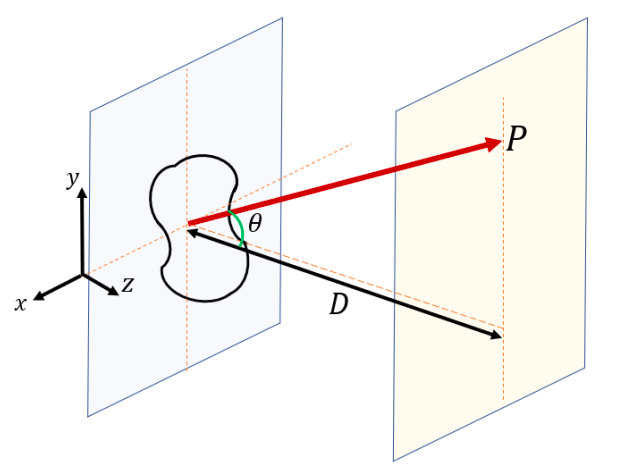Figure 1: projection of an incoming beam of light through an aperture unto a screen

The simplest possible case we can look at is the single-slit with width d and length L, as shown in Figure 2. We will consider the case in which each point on the slit acts as its own source of spherical waves. Since in practical situations the distance between the aperture and the screen is much larger than the aperture dimensions (D >> d), we can assume planar wave fronts will hit the screen. Furthermore, if we take two consecutive sources separated by a distance y as shown in Figure 3, then we can define the phase difference as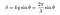Equation 1: phase difference for light waves passing through a single slit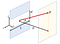Figure 2: schematic of a single slit with a finite length and width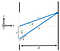Figure 3: two ray sources separated by a vertical distance y at the slit position

This is of course assuming that D>>d, which means that the wave-vectors will be almost parallel to each other. Now, assuming a planar source wave propagating along the z-axis

is incident on the aperture, every virtual source at the aperture will be described by

Now, the resulting combination of waves at any point P on the screen will be the sum of all the virtual sources we have at our aperture, namely the integral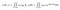Equation 2: total wave at a location z and angle θ from the horizontal

In this case, we express dS = L dy, and hence the double-integral reduces to a single-integral

At this point, we can exploit the property

to write

by way of the substitution

Now, since the source is located at a fixed distance D along the z-axis, we make the substitution

which allows us to write

and thus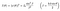Equation 3: total intensity at position z = D as a function of angle θ

with

A plot of Eq(3) as a function of β is shown in Figure 4. We can see how the center peak is much larger than every consecutive peak, and this corresponds to the region of maximum constructive interference between all the wave-fronts propagating from the slit.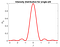Figure 4: Normalized intensity pattern from a narrow slit in 1D

# Single-slit diffraction in 2D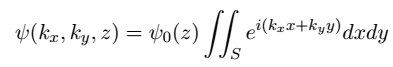Equation 4: total wave pattern from a 2-dimensional aperture perpendicular to the z-axis

In this case, we will express the field distribution ψ in terms of the wave-vector components kx and ky in order to simplify calculations. Since the domain of integration in this case is constant along both axes, and the integrand is separable, we can use the following property of double integrals (known as Fubini’s theorem)

Hence we write

Computing each integral yields

and simplifying the exponential expressions yields

with the substitutions

Finally, setting a constant C = w h ψ0(D)/4 yields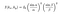Equation 5: intensity diffraction pattern from a rectangular aperture

which is plotted in Figure 5 for a rectangular aperture of size 2x2 units.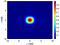Figure 5: Normalized intensity diffraction pattern from a rectangular slit of size 2x2

Oscar is a physicist, educator and STEM enthusiast. He is currently finishing a PhD in Theoretical Physics with a focus on photonics and stochastic dynamics.

## More from Oscar Nieves

Oscar is a physicist, educator and STEM enthusiast. He is currently finishing a PhD in Theoretical Physics with a focus on photonics and stochastic dynamics.